Get inspired by the success stories of our students in IIT JAM MS, ISI  MStat, CMI MSc DS.  Learn More

# ISI MStat 2016 PSA Problem 9 | Equation of a circleThis is a problem from ISI MStat 2016 PSA Problem 9 based on equation of a circle. First, try the problem yourself, then go through the sequential hints we provide.

## Equation of a circle- ISI MStat Year 2016 PSA Question 9

Given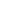in the range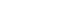the equation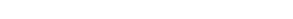represents a circle for allin the interval

•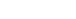•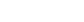••### Key Concepts

Equation of a circle

Trigonometry

Basic Inequality

Answer: isISI MStat 2016 PSA Problem 9

Precollege Mathematics

## Try with Hints

Complete the Square.

We get ,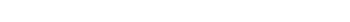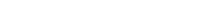We are given that. So,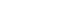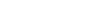.

## Subscribe to Cheenta at Youtube

This is a problem from ISI MStat 2016 PSA Problem 9 based on equation of a circle. First, try the problem yourself, then go through the sequential hints we provide.

## Equation of a circle- ISI MStat Year 2016 PSA Question 9

Givenin the rangethe equationrepresents a circle for allin the interval

••••### Key Concepts

Equation of a circle

Trigonometry

Basic Inequality

Answer: isISI MStat 2016 PSA Problem 9

Precollege Mathematics

## Try with Hints

Complete the Square.

We get ,We are given that. So,.

## Subscribe to Cheenta at Youtube

This site uses Akismet to reduce spam. Learn how your comment data is processed.

### Knowledge Partner